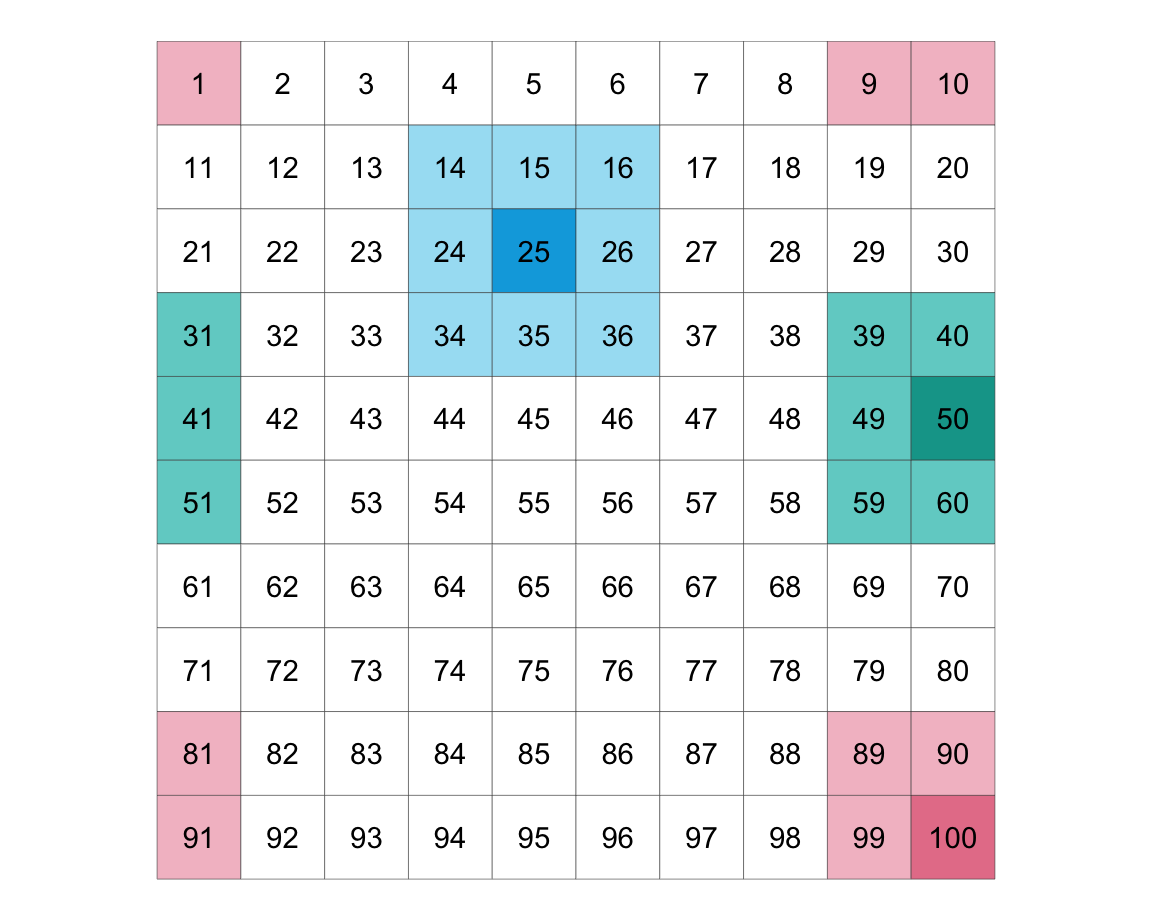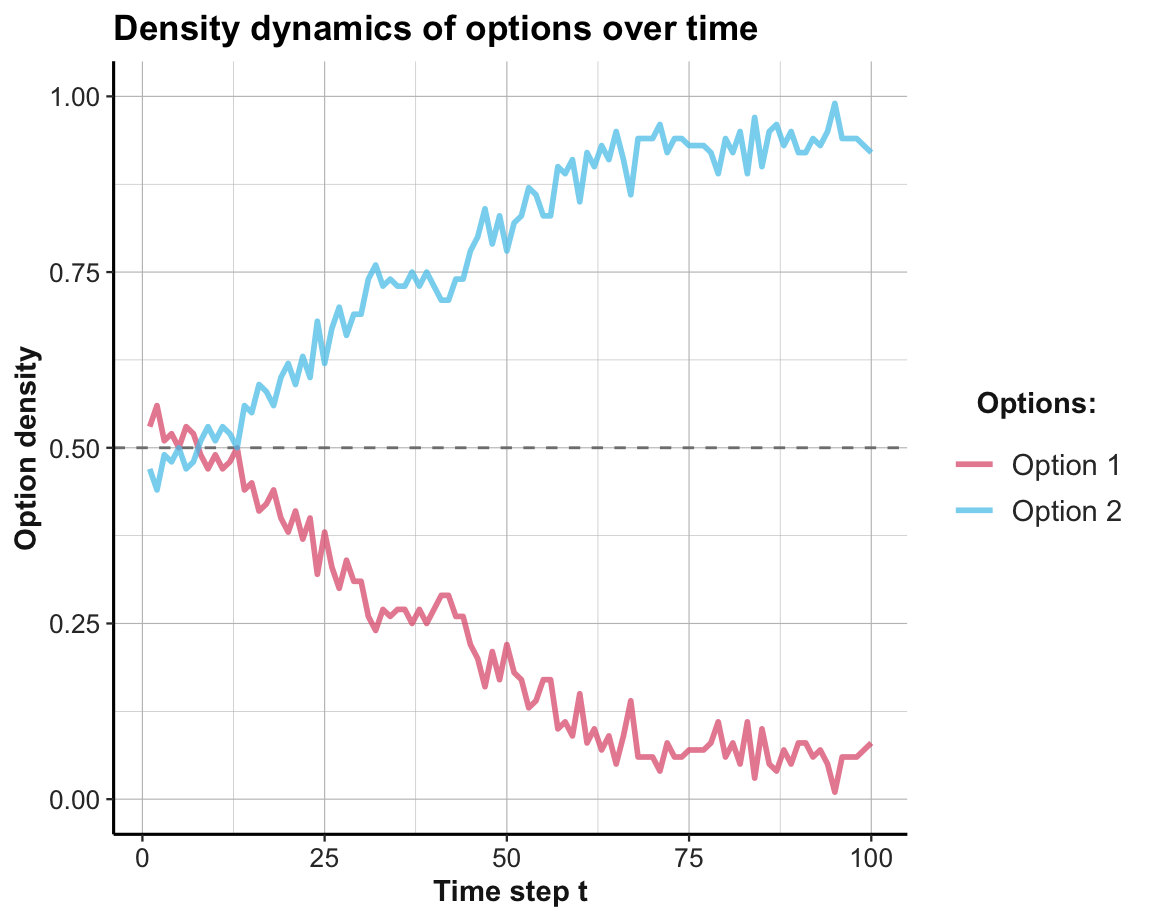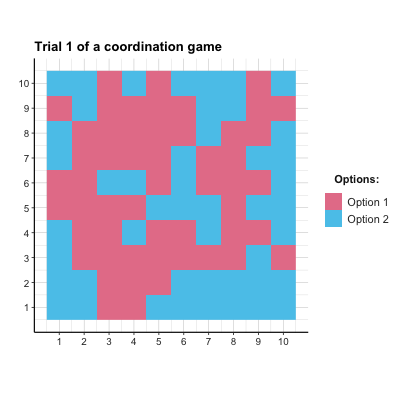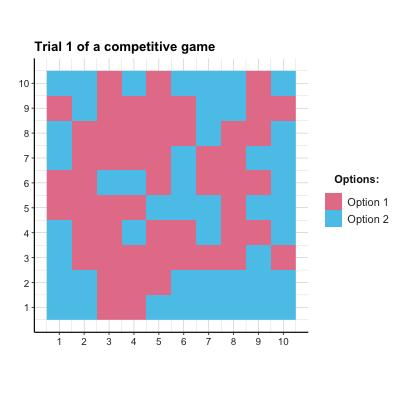## 17.2 Games

We first define what the scientific discipline of game theory means by a game.

### 17.2.1 Terminology

The scientific discipline of game theory has little resemblance to childrens’ games, but instead studies mathematical models of strategic interactions among rational decision-makers (see Wikipedia). In a similar vein, the Stanford Encyclopedia of Philosophy defines game theory as

… the study of the ways in which interacting choices of economic agents
produce outcomes with respect to the preferences (or utilities) of those agents,
where the outcomes in question might have been intended by none of the agents.

Ross (2019)

The article immediately adds that the meaning of this definition requires an understanding of the italicized concepts (and provides these details in the corresponding article). For us, it is interesting that games are expressed in terms of economic exchanges, involving agents that pursue their goals, but may obtain outcomes that also depend on the goals and actions of other agents.

We can define a game as a payoff matrix that shows the options and corresponding rewards for its players (in terms of points gained or lost for each combination of outcomes).

One of the simplest possible games is illustrated by Table 17.1. In the matching pennies (MP) game, two players each select one side of a coin (i.e., heads H, or tails T). One player wins if both sides match, the other player wins whenever both sides differ. The payoff matrix shown in Table 17.1 illustrates the options of Player 1 as rows and the first payoff value in each cell, whereas the options of Player 2 are shown as columns and the second payoff values. Thus, Player 1 wins a point ($$+1$$) and Player 2 loses a point ($$-1$$) whenever both players selected the same side of their coins (“HH” or “TT”). Player 2 wins a point ($$+1$$) and Player 1 loses a point ($$-1$$) whenever both players selected different sides of their coins (“HT” or “TH”).

Table 17.1: The payoff matrix of the matching pennies game.
Options H T
H $$(+1, -1)$$ $$(-1, +1)$$
T $$(-1, +1)$$ $$(+1, -1)$$

Winning a game can correspond to maximizing one’s individual reward in a game or to gaining more reward than other players of the same game. As the outcome of any single game may be highly dependent on chance factors, the success of a game-playing strategy is typically assessed over repeated instances of the same game. The goal of maximizing one’s own rewards can create dilemmas when contrasting different levels of aggregation (e.g., single vs. repeated games, or individual vs. population gains).

### 17.2.2 Game types

Different games can be classified into types by comparing the players’ reward functions. The most important types of games are:

1. zero-sum or pure competitive games: Some player’s wins correspond to another player’s losses, so that the total of all rewards add up to zero (0).

2. common interest, identical or symmetrical games: All players have the same reward function

3. general sum games: An umbrella category for other games (i.e., not of the other types).

Note that these categories are not mutually exclusive. For instance, the matching pennies game (shown in Table 17.1) is a symmetrical, purely competitive, zero-sum game. If one player’s win did not correspond to the other player’s loss (e.g., all payoffs of $$-1$$ were replaced by payoffs of $$0$$), the game is no longer a zero-sum game, but would still be a symmetrical, common interest game.

To provide a contrast to the competitive matching pennies game, Table 17.2 shows a coordination game. As both players still have identical payoffs, this is a symmetrical common interest game in which both players win by choosing the same side of their coins. Note that there are two different local maxima in this game: As long as both options match, no player is motivated to alter her or his choice. The notion of using the best response given the current choices of other players is an important solution concept in game theory, known as the Nash equilibrium (NE). In the coordination game defined by Table 17.2, the payoffs for TT are higher than for HH, but if a player has reasons to assume that the opponent will choose H, then selecting H is better than insisting on T.

Table 17.2: The payoff matrix of a coordination game.
Options H T
H $$(1, 1)$$ $$(0, 0)$$
T $$(0, 0)$$ $$(2, 2)$$

See , for the payoff matrices and descriptions of additional types of games. (We will address the prisoner’s dilemma, PD, and the battle of the sexes, BoS, game in Exercise 17.6.2.)

Games can be further characterized by the order of play (i.e., whether players make sequential vs. simultaneous moves) and by the challenges they pose to their players (e.g., competitive vs. coordination games). Most games assume some balanced and shared understanding of a game’s goals and rules (e.g., all players may either know their own options and payoffs, or even know the full payoff matrix). However, different games and different versions of the same game can differ substantially in the transparency of players’ actions, rewards, and strategies.

A key aspect in modeling games concerns each player’s knowledge of the goals, actions, and rewards of other players. From a modeler’s perspective, we must be aware which aspect of a potentially complex situation is being addressed by a model. This essentially boils down to asking: Which research question is being addressed by modeling this game?

### 17.2.3 Learning in games

A game can be viewed as a learning environment: A player’s task is to play the game more successfully and increase her chances of winning the game.
Given our experience in modeling dynamic agents and environments (from Chapter 16), we may not need any new tools to model strategic games. From the perspective of a player (e.g., a RL agent), the strategy and behavior of other players are part of the environment.

To do something more interesting, we will adopt a simulation created by Wataru Toyokawa (which can be found here). This will be a bit challenging, but extend our previous models in three useful ways:

1. Rather than simulating the repeated encounters of two individuals, we will simulate a spatial population of agents in a lattice/torus design.

2. Rather than using our basic learning model from Section 16.2, we implement a more sophisticated learning model: An epsilon-greedy Q learning model, which uses a softmax criterion for choosing options.

3. Given that we are simulating a spatially arranged population of agents over time, we will visualize their choices as an animation.

Methodologically, the extension from individual agents to an entire population of learning agents also motivates some 3-dimensional data structures (i.e., arrays) that provide slots for each agent/player $$p$$ and time step $$t$$).

#### Basic setup

1. Defining the game:
# Game:
N_opt <- 2  # Number of options

# Payoff matrix (per player) of the coordination game:
payoff_p1 <- matrix(c(1, 0, 0, 2), nrow = N_opt, byrow = TRUE)  # Player 1
payoff_p2 <- matrix(c(1, 0, 0, 2), nrow = N_opt, byrow = TRUE)  # Player 2

get_payoffs <- function(opt_p1, opt_p2){

# Look up values in matrices:
pay_p1 <- payoff_p1[opt_p1, opt_p2]
pay_p2 <- payoff_p2[opt_p1, opt_p2]

pay_offs <- c(pay_p1, pay_p2)

return(pay_offs)
}

## Check:
# get_payoffs(2, 2)
1. Simulation parameters:
• simulating a population of individuals, arranged in square (a so-called lattice or torus)

Basic idea: Approximating the dynamics of a large (ideally infinite) system by simulating a spatial grid of its parts.

• In each round, each individual plays against its 8 neighbors (defined as either sharing a side or a corner with the focal individual).

• Boundary condition: Individuals on the edge(s) play against those on the edge of the opposite side (bottom vs. top, left vs. right).

LAT <- 10     # length/width of lattice
N_p <- LAT^2  # population size/number of players
N_t <- 100    # number of games/rounds/time steps

# The boundary condition of the lattice/torus:
boundary_cond <- function(i) { # i is x or y coordinate:

if (i < 1) {

return (i + LAT)

} else if (i > LAT) {

return (i - LAT)

} else {

return (i)

}
}

Figure 17.1 shows the resulting structure of 100 individuals. In each round, the focal individuals in the darker (blue/green/pink) color play their eight neighbors in lighter (blue/green/pink) color.Figure 17.1: Illustrating a torus structure of 100 individuals arranged on a 10x10 lattice.

#### Consequences

The lattice/torus design creates a spatial continuum of agents, and strategies, and interactions. From the perspective of each individual agent, this setup multiplies the number of games she plays and the diversity of strategies she encounters. From the modeler’s perspective, this setup allows assessing the density of each strategy at each point in time, as well as the diffusion of strategies over time. Under some circumstances, a global pattern may emerge out of local interactions.

1. Some utility functions:
# exp_ceiling() limits the exponential of x
# to a maximum value of 2^1023:
exp_ceiling <- function(x) {

exp_x <- exp(x)  # compute exp once

ix_inf <- which(is.infinite(exp_x))  # ix of infinite values

if (length(ix_inf) == 0) {

return(exp_x)  # return as is

} else { # limit infinite values:

exp_x[ix_inf] <- 8.988466e+307  # 2^1023

return(exp_x)

}
}

## Check:
# x <- c(10, 100, 1000)  # Note: exp(710) => Inf
# exp(x)
# exp_ceiling(x)

# Simple vector calculation functions (to use in apply()):
divide_vec = function (num, den) {

return(num/den)

}

multiply_vec = function (Q, beta) {

return(Q * beta)

}
1. Set learner parameters:
# Learner:
epsilon   <- 0.1  # exploration rate (epsilon-greedy model)
alpha     <- 0.5  # learning rate/step size parameter
Q_initial <- 0    # initial payoff expectation
Q         <- array(dim = c(N_opt, N_t, N_p))
Q[ , 1, ] <- Q_initial

Note the 3-dimensional array of Q values (with dimensions of N_opt, N_t, and N_p, i.e., providing 2 x 100 x 100 slots).

1. Prepare data structures for storing intermediate results:
# Storing history:
choices       <- matrix(nrow = N_p, ncol = N_t)  # in wide format
payoffs       <- matrix(nrow = N_p, ncol = N_t)
performance   <- matrix(nrow = N_p, ncol = N_t)

choiceCounter   <- array(0, dim = c(N_opt, N_p))
riskyChoiceProb <- matrix(nrow = N_p, ncol = N_t)
netChoiceProb   <- array(dim = c(N_opt,  N_t, N_p))
netChoiceProb[ , 1, ] <- 1/N_opt

density_1 <- rep(NA, N_t)
density_2 <- rep(NA, N_t)

# this is a list where the tactics distribution of each time step will be recorded
all_choices <- list() 

#### Simulation

Running the simulation as a for loop (for each time step $$t$$):

for (t in 1:N_t) {

# Each individual chooses one option based on his/her choice probability netChoiceProb[ , t, ]:
choices[ , t] = apply(netChoiceProb[ , t, ], MARGIN = 2,
FUN = function(netChoiceProbInstance){
sample(1:N_opt, 1, prob = netChoiceProbInstance, replace = FALSE)})

all_choices$step[[t]] <- matrix(choices[ , t], nrow = LAT, ncol = LAT, byrow = TRUE) # Calculate the density of each option: density_1[t] <- which(choices[ , t] == 1) %>% length() %>% divide_vec(., LAT^2) density_2[t] <- which(choices[ , t] == 2) %>% length() %>% divide_vec(., LAT^2) # OR: # density_2[t] <- 1 - density_1[t] # Calculate payoffs of each player: # Assumption: Each player plays the game once against each of the 8 neighbors: for (p in 1:N_p) { # the position (address?) of the focal player in the lattice: i <- (p - 1) %/% LAT + 1 # quotient j <- (p - 1) %% LAT + 1 # remainder payoffs[p, t] <- 0 # initialize value for (g in c(-1, 0, 1)) { # neighbor x for (h in c(-1, 0, 1)) { # neighbor y if (g^2 + h^2 > 0) { # is FALSE iff g == h == 0, which eliminates the focal player: # Determine current payoffs (given player choices): cur_payoffs <- get_payoffs(opt_p1 = all_choices$step[[t]][i, j],
opt_p2 = all_choices$step[[t]][boundary_cond(i+g), boundary_cond(j+h)]) cur_payoff_p1 <- cur_payoffs # payoff of focal player payoffs[p, t] <- payoffs[p, t] + cur_payoff_p1 # accumulate payoffs of focal player } # if. } # for h. } # for g. } # for p. # cur_choices tracks which option was chosen by each individual on this trial: cur_choices <- choices[ , t] + N_opt * (1:N_p - 1) # Updating Q values (only if another trial is following): if (t < N_t) { # Copying the previous Q value to the current Q value: Q[ , t + 1, ] <- Q[ , t, ] QQ <- aperm(Q, c(1, 3, 2)) # transpose dimensions 2 and 3 of Q dim(QQ) <- c(N_opt * N_p, N_t) # Q value updating (note: alpha is a step-size parameter which is 1/n; the sample averaging rule): QQ[cur_choices, t + 1] <- QQ[cur_choices, t] + alpha * (payoffs[ , t] - QQ[cur_choices, t]) dim(QQ) <- c(N_opt, N_p, N_t) Q <- aperm(QQ, c(1, 3, 2)) # Softmax choice (based only on Q values): ---- Q_exp <- (Q[ , t + 1, ] * rep(20, each = N_opt)) %>% apply(., MARGIN = 2, FUN = exp_ceiling) %>% round(., 5) softmaxMatrix <- Q_exp %>% apply(1, divide_vec, den = apply(Q_exp, MARGIN = 2, FUN = sum)) %>% t() %>% round(., 5) # Softmax end. # Update choice probability matrix: ---- netMatrix <- apply(X = softmaxMatrix, MARGIN = 1, FUN = multiply_vec, beta = (1 - epsilon)) %>% t() + apply(matrix(rep(1/N_opt, N_opt * N_p), N_opt:N_p), MARGIN = 1, FUN = multiply_vec, beta = epsilon) %>% t() %>% round(., 4) netChoiceProbAperm <- aperm(netChoiceProb, c(1, 3, 2)) dim(netChoiceProbAperm) <- c(N_opt * N_p, N_t) dim(netMatrix) <- c(N_opt * N_p, 1) netChoiceProbAperm[ , t + 1] <- netMatrix dim(netChoiceProbAperm) <- c(N_opt, N_p, N_t) netChoiceProb <- aperm(netChoiceProbAperm, c(1, 3, 2)) } # if (t < N_t). } # for t. Note some assumptions: • Each player selects her choice once at the beginning of each trial. Thus, it does not consider its opponents individually. #### Results Visualizing results for a population of agents requires calculating the density of their choices, rather than individual ones. This is why we computed the density_1 and density_1 vectors during the simulation: • Density dynamics of option choices over 100 trials: # Prepare data frame: density_data <- data.frame(t = 1:N_t, density_1 = density_1, density_2 = density_2) density_long <- density_data %>% pivot_longer(cols = c(-t), names_to = 'option', values_to = 'density') ggplot(density_long) + geom_hline(yintercept = 1/N_opt, linetype = 'dashed', color = "grey50")+ geom_line(aes(t, density, color = option), size = 1, alpha = 3/4)+ scale_color_manual(values = c('density_1' = usecol(Pinky), 'density_2' = usecol(Seeblau)), labels = c("Option 1", "Option 2")) + ylim(c(0, 1)) + labs(title = "Density dynamics of options over time", x = "Time step t", y = "Option density", color = "Options:") + theme_ds4psy()#### Creating an animated plot To visualize the spatical dynamics of agent choices over time, we can show which opten each agent on the lattice/torus has chosen on every trial. This can be visualized as an animated gif, in two steps: • Prepare data: Transform the 3-dimensional array of all_choices into a 2-dimensional table all_choices_tb for (t in 1:N_t) { matrix <- as_tibble(all_choices$step[[t]], .name_repair = "unique")
names(matrix) <- as.character(1:LAT)
matrix$y <- 1:LAT if (t == 1) { all_choices_tb <- matrix %>% pivot_longer(cols = c(-y), names_to = 'x', values_to = 'option') all_choices_tb$x <- as.numeric(all_choices_tb$x) all_choices_tb$trial <- rep(t, LAT^2)

} else {

all_choices_tb_temp <- matrix %>%
pivot_longer(cols = c(-y), names_to = 'x', values_to = 'option')
all_choices_tb_temp$x <- as.numeric(all_choices_tb_temp$x)
all_choices_tb_temp\$trial <- rep(t, LAT^2)

all_choices_tb <- rbind(all_choices_tb, all_choices_tb_temp)

} # if.
}

# Check result:
dim(all_choices_tb)
#>  10000     4
#> # A tibble: 6 × 4
#>       y     x option trial
#>   <int> <dbl>  <int> <int>
#> 1     1     1      2     1
#> 2     1     2      2     1
#> 3     1     3      1     1
#> 4     1     4      1     1
#> 5     1     5      2     1
#> 6     1     6      2     1
• Create an animated image (by using the gganimate and gifski packages):
library(gganimate)
library(gifski)

animate_choices <- all_choices_tb %>%
ggplot() +
geom_raster(aes(x, y, fill = as.factor(option)))+
scale_fill_manual(values = c('1' = pal_pinky[], '2' = pal_seeblau[]),
labels = c("Option 1", "Option 2")) +
scale_x_continuous(breaks = 1:10, labels = 1:10) +
scale_y_continuous(breaks = 1:10, labels = 1:10) +
coord_equal() +
theme_ds4psy() +
# gganimate-specific parts:
transition_time(trial) +
labs(title = "Trial {frame_time} of a coordination game",
fill = "Options:", x = "", y = "") +
ease_aes("linear")

animate(animate_choices, duration = 10, fps = 10,
width = 400, height = 400, renderer = gifski_renderer())

# anim_save("images/soc_anim_coord.gif")  # save image

The resulting Figure 17.2 shows that both options are equally prominent at first, but the superior Option 2 is becoming more popular from Trial 10 onwards. Due to the agents’ tendency to explore, Option 1 is still occasionally chosen.Figure 17.2: The distribution of agent choices over time in the coordination game.

#### Practice

This exercise adjusts the simulation of the coordination game to the matching pennies (MP) game (defined by Table 17.1):

1. What is the optimal strategy that agents should learn in a purely competitive game?

2. What needs to be changed to use the above model to simulate this game?

3. Adopt the simulation to verify your answers to 1. and 2.

#### Solution

• ad 1.: As any predictable agent could be exploited, the optimal choice for an individual agent is to randomly choose options. However, it is unclear what this would mean for a population of agents arranged on a lattice/torus.

• ad 2.: Theoretically, we only need to re-define the payoff matrix of the game (shown in Table 17.1). However, as the model above yields an error (when converting negative payoffs into probabilities), we add 1 to all payoffs. This turns a zero-sum game into a competitive game with symmetrical payoffs, but has no effect on the absolute differences in payoffs that matter for our RL agents):

# Payoff matrix (per player) of a competitive (matching pennies, MP) game:
payoff_p1 <- matrix(c(1, -1, -1, 1), nrow = N_opt, byrow = TRUE) + 1  # Player 1
payoff_p2 <- matrix(c(-1, 1, 1, -1), nrow = N_opt, byrow = TRUE) + 1  # Player 2
• ad 3.: Figure 17.3 shows the result of a corresponding simulation. In this particular simulation, a slight majority of agents chose Option 1, but the population did not reach a stable equilibrium. As each agent’s optimal strategy is to choose options randomly (i.e., with a $$p=.5$$ for each option), this could be evidence for learning. But to really demonstrate successful learning, further examinations would be necessary.Figure 17.3: The distribution of agent choices over time in a competitive game.

### References

Nowé, A., Vrancx, P., & De Hauwere, Y.-M. (2012). Game theory and multi-agent reinforcement learning. In M. Wiering & M. van Otterlo (Eds.), Reinforcement learning: State-of-the-art (pp. 441–470). Springer. https://doi.org/10.1007/978-3-642-27645-3_14
Ross, D. (2019). Game theory. In E. N. Zalta (Ed.), The stanford encyclopedia of philosophy (winter 2019 edition). https://plato.stanford.edu/archives/win2019/entries/game-theory/